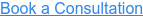How Much Tension Can You Apply to a Material Before It Breaks or Ruptures?

A tensile test, also known as tension test, is the answer to this question. In a tensile test, a specimen is subjected to a controlled tension while measuring the applied load and the elongation of the specimen. It is one of the most fundamental types of mechanical tests performed on a material. Results obtained from a tensile test are widely used to select a material for an application, for quality control, and to predict how a material will react under other types of forces.

A tensile test involves mounting the specimen in the instrument and subjecting it to tension. The tensile force is recorded as a function of the increase in gage length, which is then converted into a stress versus strain curve by taking into consideration of its dimensions. A typical stress-strain curve is shown above, from which information as follows can be obtained:

• Modulus of Elasticity
• Linear-Elastic Region
• Yield Strength point
• Ultimate Tensile Strength
• Breaking Strength
• Related Standards

• 510(k)
• ISO 10993
• USP

## Tap into the RQM+ Knowledge Center